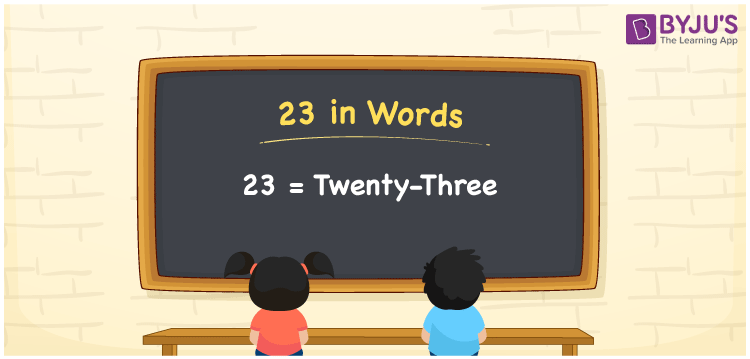# 23 in Words

We can write 23 in words as Twenty-three. If you have 23 hours left to complete a certain task, you can say, “I have only twenty-three hours left to finish this task”. However, the conversion of a cardinal number to words is simple if we use the place value chart. In this article, you can understand how to write the number 23 in words.

 23 in words Twenty-three Twenty-three in Numbers 23

## 23 in English Words

We generally write numbers in words using the English alphabet. So, we can read 23 using the words in English as “Twenty-three”.## How to Write 23 in Words?

We know that 23 is a two-digit number so let’s make a place value chart with two columns as given below.

 Tens Ones 2 3

Here, ones = 3 and tens = 2.

So, 2 × Tens + 3 × Ones

= 2 × 10 + 3 × 1

= 20 + 3

= 23

= Twenty-three

Therefore, 23 in words = Twenty-three.

### Facts About the Number 23

23 is a natural number that is the successor of 22 and the predecessor of 24.

23 in words – Twenty-three

Is 23 an even number? – No

Is 23 an odd number? – Yes

Is 23 a composite number? – No

Is 23 a prime number? – Yes

Is 23 a perfect square number? – No

Is 23 a perfect cube number? – No

How do you write 23 in Roman numerals? – XXIII

## Frequently Asked Questions on 23 in Words

Q1

### How do you write 23 in words?

In English, the spelling of 23 in words is Twenty-three, so we can write 23 in words as Twenty-three.
Q2

### Write x ≤ 23 in words.

x ≤ 23 can be written in words is “x less than or equal to twenty-three.
Q3

### How do you express 10^23 in word form?

We can express 10^23 in word form as “ten power twenty-three”.